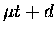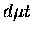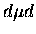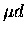Next: Condensed matter and subthreshold Up: Muonic tritium scattering Previous: The Ramsauer-Townsend effect

##scattering

From the measurements of the DS fusion yield with varying US overlayer thickness (MOD measurements), we have extracted the effective attenuation interaction length Leffatt, which agrees at the 10% level with the value given by the Monte Carlo using theelastic cross section from Ref. :

 Leffatt (exp) = (119) Leffatt (mc) = (120)

where the first error is due to the fit and the second due to the uncertainty in the US layer thickness. The attenuation length is determined mainly by theelastic scattering process, thus the agreement between Eqs. 9.1 and 9.2 suggest confirmation of thescattering cross section of Chiccoli  at the 10% level. This is the first quantitative measurement of thecross section to our knowledge, as conventional cycling measurements in D/T mixtures are not directly sensitive to this process. We note that dependence of our results on the value offormation rate is rather weak; a change of by a factor of 2 produces variations in Leffatt similar to or smaller than those due to the thickness uncertainty.

Our data could alternatively be used to extract information on the scattering angular distribution. A MC calculation assuming isotropic scattering with the same total cross section as Ref.  resulted in the effective attenuation length:

 Leffatt (mc,iso) = (121)

which is in clear disagreement with our experiment. Theelastic cross sections are in fact predicted to have large p-wave contributions, whose angular distribution is strongly peaked forward . This is due to the existence of the loosely boundmolecular state with an angular momentum of J=1, the very state responsible for the resonant molecular formation. The energy loss of a particle in the lab frame in an elastic collision is uniquely determined by the scattering angle . A scattering to large angles will reduce the energy substantially (depending on the masses involved), while the particles do not suffer much energy loss for forward angle scattering. Hence, the forward peaked angular distribution results in a smaller attenuation, compared to the isotropic one for the same total cross section.

In order to reproduce our experimental attenuation length Leffintusing the isotropic angular distribution, the total cross section needs to be half of the predicted value, which seems rather unlikely (the experimental absolute fusion yield at US thickness zero is reasonably well reproduced by either model). Thus, our results given in Table 8.15 confirm the importance of the p-wave contribution inscattering.

Furthermore, the suggestion of the large p-wave contribution in turn provides indirect yet intriguing experimental evidence for the existence of the J=1 state near thethreshold. Note that while for themolecule there is some evidence for its J state from the fusion branching ratio of the two channels (it is predicted to be sensitive to J according to R-matrix calculations ), there has been little direct experimental information of the J state of the loosely bound.

Finally, our analysis presented here suggests that the measurement by Strasser et al. , who assumed an isotropic angular distribution in the simulation of thedeceleration in deuterium, may require re-interpretation. Since there is a significant p-wave contribution also in the interaction, neglecting its angular distribution is not justified, as demonstrated in our analysis. Therefore, their conclusion, which stressed the importance of molecular effects, should be taken with caution.Next: Condensed matter and subthreshold Up: Muonic tritium scattering Previous: The Ramsauer-Townsend effect